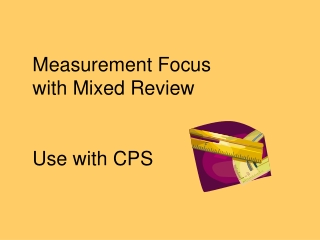# Measurement Focus with Mixed Review Use with CPS - PowerPoint PPT PresentationDownload PresentationMeasurement Focus with Mixed Review Use with CPS

Measurement Focus with Mixed Review Use with CPS
Download Presentation## Measurement Focus with Mixed Review Use with CPS

- - - - - - - - - - - - - - - - - - - - - - - - - - - E N D - - - - - - - - - - - - - - - - - - - - - - - - - - -
##### Presentation Transcript

1. Measurement Focus with Mixed Review Use with CPS

2. Which is the closest length of the chain pictured above. Use your graphics sheet and ruler to measure. • 2 5/8 inches • 3 5/8 inches • 6 ½ inches • 7 ½ inches

3. A quart is almost a _______________. • Milliliter • Centiliter • Liter • Kiloliter

4. Which appears to be a pair of congruent figures?

5. Anna bought one pound of butter. Which of the following is equivalent to 1 pound. • 10 ounces • 16 ounces • 28 ounces • 36 ounces

6. A school bus picks up 54 students each day.  The distance each student lives from school is shown on the graph below.  How many students live 8 or more miles from school? A  13 B  14 C  27 D  29

7. If the mass of the 5 nickels is 25 grams, which measurement is closest to the total mass of the 2 pieces of candy? A 35 grams B 30 grams C 25 grams D 20 grams

8. Line m and four points are shown on the grid. Which two points appear to lie on the same line that is parallel to line m? A G and D B D and E C G and E D F and D

9. Which of the following is closest to the height of the bookmark shown? A. 3 inches B. 3 ¼ inches C. 3 ¾ inches D. 4 inches

10. Josh measured four pieces of string that were different colors.  His results are shown in the table below.  Which list of colors shows the pieces of string in order from shortest to longest? A.  Yellow, Green, Red, Orange B.  Red, Green, Orange, Yellow C.  Yellow, Orange, Green, Red D.  Orange, Red, Green, Yellow

11. Elsa made the graph below to show the amount of money her family spent on food each week for one month. Between which two weeks was the difference in the amount spent on food closest to \$5? ABetween week 1 and week 2 B Between week 2 and week 3 C Between week 1 and week 4 D Between week 2 and week 4

12. The line graph shows how Joseph’s height changed as he grew. Which of the following is closest to Joseph’s height when he was 15 years old? A 80 in. B 70 in. C 65 in. D 60 in.

13. Dan is buying packages of cookies for class. Each package of cookies contains the same number of cookies. Based on the information from the table, how many packages will Dan need to buy to have a total of 32 cookies? A 6 B 7 C 8 D 9

14. Which product is between 550 and 600 ? A. 52×12 B. 45×12 C. 56×12 D. 48×12

15. Mr. Guzman is making a bookcase. Each shelf will be 1 yard long. A length of 1 yard is about the same as — A 10 millimeters B 10 centimeters C 1 kilometer D 1 meter

16. Joe ran a 100-meter race. How many centimeters are equal to 100 meters? A 10 B 100 C 1,000 D 10,000

17. Josie is mailing a package that weighs about two pounds.  What could be in the package? A  a necklace B  a cell phone C  a pair of jeans D  a car tire

18. Look at the tape that has been rolled off the roll.  What is the best estimate of the length? A.  4 yards B.  4 meters C.  4 inches D.  4 centimeters

19. What do a ray and a line segment have in common? A Both have two endpoints. B Both are part of a line. C Both go on and on infinitely. D Both include only 10 points

20. Mr. Zander bought 1 pint of chocolate milk. Which of the following is equivalent to 1 pint? A ½ cup B 1 quart C 2 cups D gallon

21. Mr. Yung cuts several boards so that they will all be 54 inches long.  Which of the following measurements is equal to 54 inches? A   4 1/2 feet B   5 1/2 feet C   4 feet 4 inches D   5 feet 4 inches

22. Troy dropped some craft sticks on the floor as shown in the picture. Which two craft sticks are best described as intersecting but not perpendicular? A 2 and 3 B 2 and 1 C 4 and 3 D 4 and 1

23. This graph shows the total amount of electricity used by a 75-watt light bulb in constant use.  Which is the closest to the number of watts the light bulb will have used after being on for 16 hours? A.  800 B.  1,000 C.  1,200 D.  1,400

24. Marcy’s scrapbook is 12 inches wide. Which of the following is equivalent to 12 inches? A 1 yard B 1 foot C 1 centimeter D 1 meter

25. Which is true? A. 4×9=9÷4 B. 4×9=9−4 C. 4×9=9×4 D. 4×9=9+4

26. Jessica is making a necklace with beads like the one shown below.  Use your centimeter ruler to  help you estimate the best measure of the line segment under the bead.   About how long is one bead? A  2 mm B  2 cm C  8 mm D  8 cm